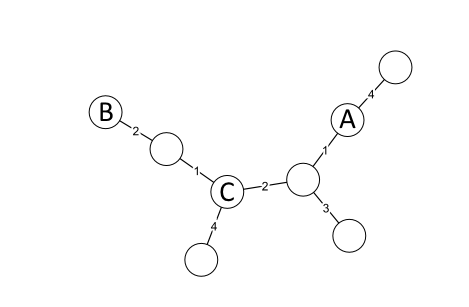# Minimum Spanning Trees

A minimum spanning tree is a tree connecting all nodes in a graph, in a way such that the sum of edge lengths is minimized, see figure 12.5.Figure 12.5: Minimum Spanning Tree construction. (Public domain image from Wikimedia: Minimum spanning tree.svg.)Figure 12.6: Minimum Spanning Tree distances.

Minimum spanning trees can be a bit counter-intuitive. Consider figure 12.6: The distance between A and B is not necessarily larger than the distance from A to C. But we do know that the distance between two nodes is greater or equal to the largest edge connecting them. (e.g. the distance between A and B is at least two, and the distance between A and C is at least two)

Minimum spanning trees are often used to visualize relationships between strains or isolates. But note that MST's are not unique - there are often many possible trees, especially for the classic 7-gene schemes, where there are only a very limited number of possible edge lengths. In order to break the ties when constructing the tree, our MST implementation favor creating connections to nodes that have many low-distance relations in the allelic distance matrix.

Minimum Spanning Trees can be created using the Create MLST Scheme, the Download MLST Scheme, the Import MLST Scheme tools or the Create MLST Sub Scheme button of an existing scheme.

Subsections